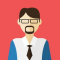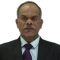## What is "is not" operator in Python?Published on 11-Feb-2018 19:09:21
In Python is not membership operator evaluates to false if the variables on either side of the operator point to the same object and true otherwise. For example − >>> a = 10 >>> b = 20 >>> id(a), id(b) (1581561184, 1581561504) >>> a is not b True ... Read More

## What is "not in" operator in Python?Published on 11-Feb-2018 19:14:40
In Python 'not in' membership operator evaluates to true if it does not finds a variable in the specified sequence and false otherwise. For example >>> a = 10 >>> b = 4 >>> l1 = [1, 2, 3, 4, 5] >>> a not in l1 True >>> b ... Read More

## What are different bitwise operators types in Python?Published on 11-Feb-2018 19:32:23
Bitwise operators operate upon bits as operands. Following bitwise operators are defined in Python − & (bitwise AND): returns 1 if both bit operands are 1 | (bitwise OR): returns 1 even if one of two bit operands is 1 ^ (bitwise XOR): returns 1 only if one operand ... Read More

## What are different assignment operators types in Python?Published on 11-Feb-2018 19:42:11
Following table shows all assignment operators − Operator Description Example = Assigns values from right side operands to left side operand c = a + b value of a + b into c += It adds right operand to the left operand and ... Read More

## What are different Identity operators types in Python?Published on 11-Feb-2018 19:50:38
Each Python object is assigned a unique identification number when it is stored in memory. It can be fetched by id() function. The is operator compares id() of two objects and returns True if both objects have same value otherwise it returns false. The is not operator on the other ... Read More

## What is correct operators precedence in Python?Published on 10-Feb-2018 08:34:19
Following table shows order of precedence of operators in Python starting from highest to lowest precedence.** : Exponentiation (raise to the power)~ + - : Ccomplement, unary plus and minus (method names for the last two are +@ and -@)* / % // : Multiply, divide, modulo and floor division+ ... Read More

## How does ++ and -- operators work in Python?Published on 10-Feb-2018 08:26:58
In C, C++, Java etc ++ and -- operators increment and decrement value of a variable by 1. In Python these operators won't work. In Python variables are just labels to objects in memory. In Python numeric objects are immutable. Hence by a++ (if a=10) we are trying to increment value ... Read More

## Does Python have a ternary conditional operator?Published on 10-Feb-2018 08:13:17
Ternary operator was added in Python 2.5. Its syntax is:Syntaxx if expr==True else yExampleFollowing example illustrates usage>>> percent=59 >>> 'pass' if percent>=50 else 'fail' 'pass' >>> percent=45 >>> 'pass' if percent>=50 else 'fail' 'fail'

## What are different arithmetic operators in Python?Published on 10-Feb-2018 08:05:28
Arithmetic operators in Python are explained below −+ for addition − performs addition of operands on either side>>a=5 >>b=7 >>c=a+b >>c 12- for subtraction − subtracts second operand from first>>a=10 >>b=5 >>c=a-b >>c 5* for multiplication − multiplies first operand by second>>a=5 >>b=2 >>c=a*b >>c 10/ for division − divides ... Read More

## What are different basic operators in Python?Published on 10-Feb-2018 07:56:37
Operators in Python are classified as −Arithmetic operators+ for addition- for subtraction* for multiplication/ for division// for floor division% for modulo or remainderRelational operators> for greater than>= for greater than or equal to< for less than<= for less than or equal to== for is equal to!= for is not equal ... Read More